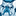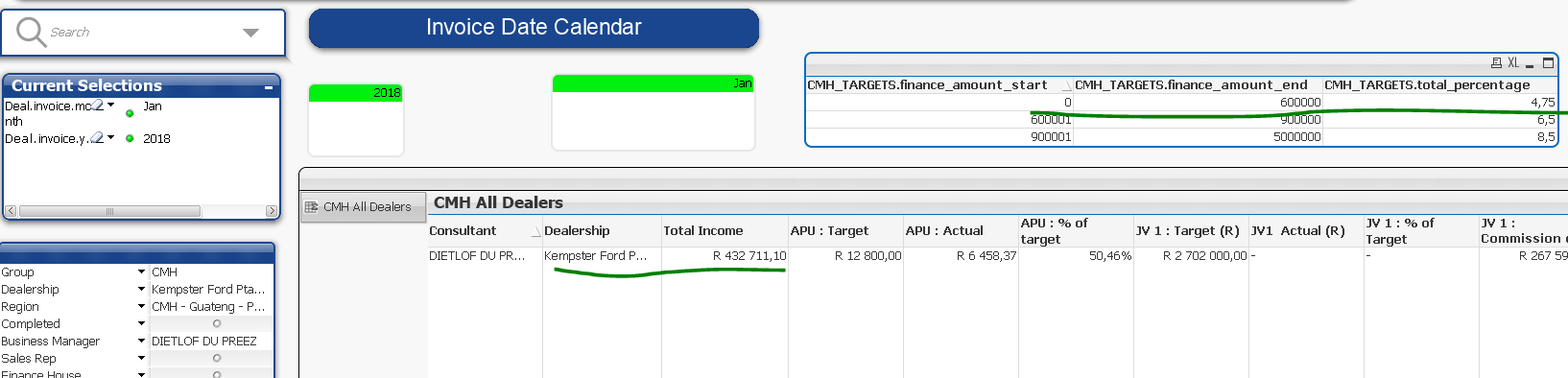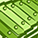# QlikView App Dev

Discussion Board for collaboration related to QlikView App Development.

Announcements
cancel
Showing results for
Did you mean:Creator III

## How to get the correct total_percentage ??

Hi All ,

Please kindly assist me to create the script with the intervalmatch().

I am providing the sample model.

The requirement :

According , to the Total Income of Dealership , the total_percentage should pick.

Say , For eg ,

The dealership : Kempster Ford Pta New

Total Income : 432711

Thus , It should lie between finance_amount_start (0) and finance_amount_end (600000)

and the total_percentage should be 4.75.

How , can I store the total_percentage in variable . As , I need to use the total_percentage for Commision Calculation

depending on the Total Income lies in which level.

0-600000

600000-900000

900000-50000001 Solution

Accepted SolutionsCreator

varPercentage =

sum

({

\$<CMH_TARGETS.finance_amount_start = {"<= \$(=\$(vCmhTotalIncome))"},

CMH_TARGETS.finance_amount_end = {">= \$(=\$(vCmhTotalIncome))"}>

}

CMH_TARGETS.total_percentage

)

6 RepliesCreator

varPercentage = if ( \$(#vCmhTotalIncome) >=0 and \$(#vCmhTotalIncome)

<= 600000,4.75)Creator III
Author

I have already tried to use

if ( \$(#vCmhTotalIncome) >=0 and \$(#vCmhTotalIncome)<= 600000,CMH_TARGETS.total_percentage)

As the total_percentage is dynamic , The cant state as 4.75.

But , with my expression , the variable is picking all the three values , 4.75,6.5,8.5

Thanks,Partner

Hi,

Try below

if ( \$(#vCmhTotalIncome) >=0 and \$(#vCmhTotalIncome)

<= 600000,CMH_TARGETS.total_percentage,if ( \$(#vCmhTotalIncome) >=600001 and \$(#vCmhTotalIncome)

<= 900000,CMH_TARGETS.total_percentage,if ( \$(#vCmhTotalIncome) >=900001 and \$(#vCmhTotalIncome)

<= 5000000,CMH_TARGETS.total_percentage)))Creator III
Author

Sorry , It didn't worked.Creator

varPercentage =

sum

({

\$<CMH_TARGETS.finance_amount_start = {"<= \$(=\$(vCmhTotalIncome))"},

CMH_TARGETS.finance_amount_end = {">= \$(=\$(vCmhTotalIncome))"}>

}

CMH_TARGETS.total_percentage

)Creator III
Author

Hi RODELL BASALO ,

It worked partially ,

The Total Percentage is showing 7 as the Total Income is doing the total sum of Total Income ,

Due to Sum of Total Income = 621914 is between 600001 - 900000 thus showing 7.

But my requirement is , the percentage should vary of every row of Total Income.

Say For eg ,

For Total Income 6999 the total percentage is 5

For Total Income 598739 the total percentage is 5

For Total Income 16175 the total percentage is 5

If for eg , there is any Total Income - 869000 then the total percentage should be 7

and Total Income - 950000 then the total percentage should be 6

sample model attached. Please assist. Thanks,